# Scopes by example

#### SSharp S# API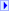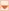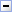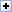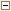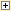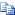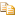Creating global variable

a = 1;

Creating global variable in local scope

//Global scope
{ //Local scope 1
{//Local scope 2
//Create global variable from local scope
a = 4;
}
}
// In this scope a = 4
return a;

Temporary local variables

//Global scope
{ //Local scope 1
var a; //Create empty variable in local scope 1
{//Local scope 2
//This will set variable to top-most scope which contains
//definition for variable, which is Local scope 1
a = 4;
}
}
//Global scope still empty

Variable resolving rules

//Global scope
{ //Local scope 1
var a; //Create empty variable in local scope 1
{//Local scope 2
//This will set variable to top-most scope which contains
//definition for variable, which is Local scope 1
a = 5;
{ //Local scope 3
var a; //Create empty variable in local scope 3
//This will set variable to top-most scope which contains
//definition for variable, which is Local scope 1
global:a = 4;
a = 3; // Set local variable
}
}
//Create variable in global scope equal to current value of a in this scope
b = a;
}
//b = 4;
return b;

Temporary variables in for loop

var sum = 0;
for (var x=0; x<10; x++){
var temp = x;
sum += x;
}
//Here temp and x variables are absent
return sum;